# Lorene::Ideal_gas Class Reference [Equations of state]

Ideal-gas (temperature-dependent) equation of state, with mass-term in the energy density. More...

#include <hoteos.h>

Inheritance diagram for Lorene::Ideal_gas: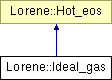List of all members.

## Public Member Functions

Ideal_gas (double gamma, double kappa, double mass=1.)
Standard constructor.
Ideal_gas (const Ideal_gas &)
Copy constructor.
virtual ~Ideal_gas ()
Destructor.
void operator= (const Ideal_gas &)
Assignment to another Ideal_gas.
virtual bool operator== (const Hot_eos &) const
Comparison operator (egality).
virtual bool operator!= (const Hot_eos &) const
Comparison operator (difference).
virtual int identify () const
Returns a number to identify the sub-classe of Hot_eos the object belongs to.
double get_gam () const
Returns the adiabatic index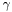(cf. Eq. (1)).
double get_kap () const
Returns the pressure coefficient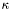(cf. Eq. (1)).
double get_m_0 () const
Return the individual particule mass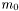(cf.
virtual const Eosnew_cold_Eos () const
Returns the corresponding cold Eos.
virtual void sauve (FILE *) const
Save in a file.
virtual double nbar_Hs_p (double ent, double sb) const
Computes the baryon density from the log-enthalpy and entropy per baryon (virtual function implemented in the derived classes).
virtual double ener_Hs_p (double ent, double sb) const
Computes the total energy density from the log-enthalpy and entropy per baryon (virtual function implemented in the derived classes).
virtual double press_Hs_p (double ent, double sb) const
Computes the pressure from the log-enthalpy and entropy per baryon (virtual function implemented in the derived classes).
virtual double temp_Hs_p (double ent, double sb) const
Computes the temperature from the log-enthalpy and entropy per baryon (virtual function implemented in the derived classes).
const string & get_name () const
Returns the hot EOS name.
void set_name (const char *)
Sets the hot EOS name.
virtual bool operator== (const Hot_eos &) const =0
Comparison operator (egality).
virtual bool operator!= (const Hot_eos &) const =0
Comparison operator (difference).
Scalar nbar_Hs (const Scalar &ent, const Scalar &sb, int nzet, int l_min=0) const
Computes the baryon density field from the log-enthalpy field and entropy per baryon.
Scalar ener_Hs (const Scalar &ent, const Scalar &sb, int nzet, int l_min=0) const
Computes the total energy density from the log-enthalpy and entropy per baryon.
Scalar press_Hs (const Scalar &ent, const Scalar &sb, int nzet, int l_min=0) const
Computes the pressure from the log-enthalpy and entropy per baryon.
Scalar temp_Hs (const Scalar &ent, const Scalar &sb, int nzet, int l_min=0) const
Computes the temperature field from the log-enthalpy field and entropy per baryon.

## Static Public Member Functions

static Hot_eoshoteos_from_file (FILE *)
Construction of an EOS from a binary file.
static Hot_eoshoteos_from_file (ifstream &)
Construction of a hot EOS from a formatted file.

## Protected Member Functions

Ideal_gas (FILE *)
Constructor from a binary file (created by the function sauve(FILE*) ).
Ideal_gas (ifstream &)
Constructor from a formatted file.
void set_auxiliary ()
Computes the auxiliary quantities gam1 , unsgam1 , gam1sgamkap from the values of gam and kap.
virtual ostream & operator>> (ostream &) const
Operator >>.
virtual void del_deriv () const
Deletes all the derived quantities.
void set_der_0x0 () const
Sets to 0x0 all the pointers on derived quantities.
void calcule (const Scalar &thermo1, const Scalar &thermo2, int nzet, int l_min, double(Hot_eos::*fait)(double, double) const, Scalar &resu) const
General computational method for Scalar 's.

## Protected Attributes

double gam
Adiabatic index.
double kap
Pressure coefficient(cf.
double m_0
Individual particule mass(cf.
double gam1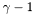double unsgam1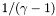double gam1sgamkap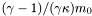string name
EOS name.
Eosp_cold_eos
Corresponding cold Eos.

## Friends

Hot_eosHot_eos::hoteos_from_file (FILE *)
The construction functions from a file.
Hot_eosHot_eos::hoteos_from_file (ifstream &)
ostream & operator<< (ostream &, const Hot_eos &)
Display.

## Detailed Description

Ideal-gas (temperature-dependent) equation of state, with mass-term in the energy density.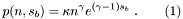and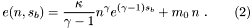### (to be written...)

()

Definition at line 362 of file hoteos.h.

## Constructor & Destructor Documentation

 Lorene::Ideal_gas::Ideal_gas ( double gamma, double kappa, double mass = 1. )

Standard constructor.

Unless specified, the individual particle massis set to the mean baryon mass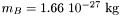.

Parameters:
 gamma adiabatic indexkappa pressure coefficientmass individual particule massDefinition at line 67 of file ideal_gas.C.

References set_auxiliary().

 Lorene::Ideal_gas::Ideal_gas ( const Ideal_gas & eosi )

Copy constructor.

Definition at line 76 of file ideal_gas.C.

References set_auxiliary().

 Lorene::Ideal_gas::Ideal_gas ( FILE * fich )  [protected]

Constructor from a binary file (created by the function sauve(FILE*) ).

This constructor is protected because any hot EOS construction from a binary file must be done via the function Hot_eos::eos_from_file(FILE*) .

Definition at line 85 of file ideal_gas.C.

References Lorene::fread_be(), gam, kap, m_0, and set_auxiliary().

 Lorene::Ideal_gas::Ideal_gas ( ifstream & fich )  [protected]

Constructor from a formatted file.

This constructor is protected because any EOS construction from a formatted file must be done via the function Hot_eos::hoteos_from_file(ifstream&) .

Definition at line 99 of file ideal_gas.C.

References gam, kap, m_0, and set_auxiliary().

 Lorene::Ideal_gas::~Ideal_gas ( )  [virtual]

Destructor.

Definition at line 113 of file ideal_gas.C.

## Member Function Documentation

 void Lorene::Hot_eos::calcule ( const Scalar & thermo1, const Scalar & thermo2, int nzet, int l_min, double(Hot_eos::*)(double, double) const fait, Scalar & resu ) const [protected, inherited]

General computational method for Scalar 's.

Parameters:
 thermo1 [input] first thermodynamical quantity (for instance the enthalpy field) from which the thermodynamical quantity resu is to be computed. thermo2 [input] second thermodynamical quantity (for instance the entropy field) from which the thermodynamical quantity resu is to be computed. nzet [input] number of domains where resu is to be computed. l_min [input] index of the innermost domain is which resu is to be computed [default value: 0]; resu is computed only in domains whose indices are in [l_min,l_min+nzet-1] . In the other domains, it is set to zero. fait [input] pointer on the member function of class Hot_eos which performs the pointwise calculation. resu [output] result of the computation.

Definition at line 153 of file hoteos.C.

 void Lorene::Hot_eos::del_deriv ( ) const [protected, virtual, inherited]

Deletes all the derived quantities.

Definition at line 109 of file hoteos.C.

References Lorene::Hot_eos::p_cold_eos, and Lorene::Hot_eos::set_der_0x0().

 Scalar Lorene::Hot_eos::ener_Hs ( const Scalar & ent, const Scalar & sb, int nzet, int l_min = 0 ) const [inherited]

Computes the total energy density from the log-enthalpy and entropy per baryon.

Parameters:
 ent [input, unit: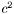] log-enthalpy H defined by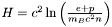, where e is the (total) energy density, p the pressure, n the baryon density, and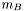the baryon mass sb [input, unit: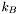] entropy per baryon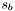nzet number of domains where the energy density is to be computed. l_min index of the innermost domain is which the energy density is to be computed [default value: 0]; the energy density is computed only in domains whose indices are in [l_min,l_min+nzet-1] . In the other domains, it is set to zero.
Returns:
energy density [unit: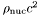], where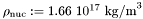Definition at line 252 of file hoteos.C.

 double Lorene::Ideal_gas::ener_Hs_p ( double ent, double sb ) const [virtual]

Computes the total energy density from the log-enthalpy and entropy per baryon (virtual function implemented in the derived classes).

Parameters:
 ent [input, unit:] log-enthalpy H defined by, where e is the (total) energy density, p the pressure, n the baryon density, andthe baryon mass sb [input, unit:] entropy per baryonReturns:
energy density e [unit:], whereImplements Lorene::Hot_eos.

Definition at line 266 of file ideal_gas.C.

References Lorene::exp(), gam, gam1, gam1sgamkap, kap, m_0, Lorene::pow(), and unsgam1.

 double Lorene::Ideal_gas::get_gam ( ) const

Returns the adiabatic index(cf. Eq. (1)).

Definition at line 146 of file ideal_gas.C.

References gam.

 double Lorene::Ideal_gas::get_kap ( ) const

Returns the pressure coefficient(cf. Eq. (1)).

Definition at line 150 of file ideal_gas.C.

References kap.

 double Lorene::Ideal_gas::get_m_0 ( ) const

Return the individual particule mass(cf.

Eq. (1))

Definition at line 154 of file ideal_gas.C.

References m_0.

 const string& Lorene::Hot_eos::get_name ( ) const [inline, inherited]

Returns the hot EOS name.

Definition at line 120 of file hoteos.h.

References Lorene::Hot_eos::name.

 Hot_eos * Lorene::Hot_eos::hoteos_from_file ( ifstream & fich )  [static, inherited]

Construction of a hot EOS from a formatted file.

The fist line of the file must start by the EOS number, according to the following conventions:

• 1 = relativistic ideal gas (class Ideal_gas ).
• 2 = non-relativistic ideal gas (class Ideal_gas_norel ).

The second line in the file should contain a name given by the user to the EOS. The following lines should contain the EOS parameters (one parameter per line), in the same order than in the class declaration.

Definition at line 107 of file hoteos_from_file.C.

 Hot_eos * Lorene::Hot_eos::hoteos_from_file ( FILE * fich )  [static, inherited]

Construction of an EOS from a binary file.

The file must have been created by the function sauve(FILE*) .

Definition at line 70 of file hoteos_from_file.C.

 int Lorene::Ideal_gas::identify ( ) const [virtual]

Returns a number to identify the sub-classe of Hot_eos the object belongs to.

Implements Lorene::Hot_eos.

Definition at line 61 of file hoteos_from_file.C.

 Scalar Lorene::Hot_eos::nbar_Hs ( const Scalar & ent, const Scalar & sb, int nzet, int l_min = 0 ) const [inherited]

Computes the baryon density field from the log-enthalpy field and entropy per baryon.

Parameters:
 ent [input, unit:] log-enthalpy H defined by, where e is the (total) energy density, p the pressure, n the baryon density, andthe baryon mass sb [input, unit:] entropy per baryonnzet number of domains where the baryon density is to be computed. l_min index of the innermost domain is which the baryon density is to be computed [default value: 0]; the baryon density is computed only in domains whose indices are in [l_min,l_min+nzet-1] . In the other domains, it is set to zero.
Returns:
baryon density [unit: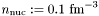]

Definition at line 236 of file hoteos.C.

 double Lorene::Ideal_gas::nbar_Hs_p ( double ent, double sb ) const [virtual]

Computes the baryon density from the log-enthalpy and entropy per baryon (virtual function implemented in the derived classes).

Parameters:
 ent [input, unit:] log-enthalpy H defined by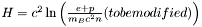, where e is the (total) energy density, p the pressure, n the baryon density, andthe baryon mass sb [input, unit:] entropy per baryonReturns:
baryon density [unit:]

Implements Lorene::Hot_eos.

Definition at line 253 of file ideal_gas.C.

References Lorene::exp(), gam1sgamkap, Lorene::pow(), and unsgam1.

 const Eos & Lorene::Ideal_gas::new_cold_Eos ( ) const [virtual]

Returns the corresponding cold Eos.

Implements Lorene::Hot_eos.

Definition at line 163 of file ideal_gas.C.

References gam, kap, m_0, and Lorene::Hot_eos::p_cold_eos.

 virtual bool Lorene::Hot_eos::operator!= ( const Hot_eos & ) const [pure virtual, inherited]

Comparison operator (difference).

 bool Lorene::Ideal_gas::operator!= ( const Hot_eos & eos_i ) const [virtual]

Comparison operator (difference).

Definition at line 215 of file ideal_gas.C.

References operator==().

 void Lorene::Ideal_gas::operator= ( const Ideal_gas & eosi )

Assignment to another Ideal_gas.

Definition at line 119 of file ideal_gas.C.

References gam, kap, m_0, Lorene::Hot_eos::name, and set_auxiliary().

 virtual bool Lorene::Hot_eos::operator== ( const Hot_eos & ) const [pure virtual, inherited]

Comparison operator (egality).

 bool Lorene::Ideal_gas::operator== ( const Hot_eos & eos_i ) const [virtual]

Comparison operator (egality).

Definition at line 178 of file ideal_gas.C.

References gam, identify(), Lorene::Hot_eos::identify(), kap, and m_0.

 ostream & Lorene::Ideal_gas::operator>> ( ostream & ost ) const [protected, virtual]

Operator >>.

Implements Lorene::Hot_eos.

Definition at line 234 of file ideal_gas.C.

References gam, kap, and m_0.

 Scalar Lorene::Hot_eos::press_Hs ( const Scalar & ent, const Scalar & sb, int nzet, int l_min = 0 ) const [inherited]

Computes the pressure from the log-enthalpy and entropy per baryon.

Parameters:
 ent [input, unit:] log-enthalpy H defined by, where e is the (total) energy density, p the pressure, n the baryon density, andthe baryon mass sb [input, unit:] entropy per baryonnzet number of domains where the pressure is to be computed. l_min index of the innermost domain is which the pressure is to be computed [default value: 0]; the pressure is computed only in domains whose indices are in [l_min,l_min+nzet-1] . In the other domains, it is set to zero.
Returns:
pressure [unit:], whereDefinition at line 266 of file hoteos.C.

 double Lorene::Ideal_gas::press_Hs_p ( double ent, double sb ) const [virtual]

Computes the pressure from the log-enthalpy and entropy per baryon (virtual function implemented in the derived classes).

Parameters:
 ent [input, unit:] log-enthalpy H defined by, where e is the (total) energy density, p the pressure, n the baryon density, andthe baryon mass sb [input, unit:] entropy per baryonReturns:
pressure p [unit:], whereImplements Lorene::Hot_eos.

Definition at line 282 of file ideal_gas.C.

References Lorene::exp(), gam, gam1, gam1sgamkap, kap, Lorene::pow(), and unsgam1.

 void Lorene::Ideal_gas::sauve ( FILE * fich ) const [virtual]

Save in a file.

Reimplemented from Lorene::Hot_eos.

Definition at line 224 of file ideal_gas.C.

References Lorene::fwrite_be(), gam, kap, and m_0.

 void Lorene::Ideal_gas::set_auxiliary ( )  [protected]

Computes the auxiliary quantities gam1 , unsgam1 , gam1sgamkap from the values of gam and kap.

Definition at line 136 of file ideal_gas.C.

References gam, gam1, gam1sgamkap, kap, m_0, and unsgam1.

 void Lorene::Hot_eos::set_der_0x0 ( ) const [protected, inherited]

Sets to 0x0 all the pointers on derived quantities.

Definition at line 114 of file hoteos.C.

References Lorene::Hot_eos::p_cold_eos.

 void Lorene::Hot_eos::set_name ( const char * name_i )  [inherited]

Sets the hot EOS name.

Definition at line 118 of file hoteos.C.

References Lorene::Hot_eos::name.

 Scalar Lorene::Hot_eos::temp_Hs ( const Scalar & ent, const Scalar & sb, int nzet, int l_min = 0 ) const [inherited]

Computes the temperature field from the log-enthalpy field and entropy per baryon.

Parameters:
 ent [input, unit:] log-enthalpy H defined by, where e is the (total) energy density, p the pressure, n the baryon density, andthe baryon mass sb [input, unit:] entropy per baryonnzet number of domains where the baryon density is to be computed. l_min index of the innermost domain is which the baryon density is to be computed [default value: 0]; the baryon density is computed only in domains whose indices are in [l_min,l_min+nzet-1] . In the other domains, it is set to zero.
Returns:
temperature [unit: MeV]

Definition at line 280 of file hoteos.C.

 double Lorene::Ideal_gas::temp_Hs_p ( double ent, double sb ) const [virtual]

Computes the temperature from the log-enthalpy and entropy per baryon (virtual function implemented in the derived classes).

Parameters:
 ent [input, unit:] log-enthalpy H defined by, where e is the (total) energy density, p the pressure, n the baryon density, andthe baryon mass sb [input, unit:] entropy per baryonReturns:
temperature [unit: MeV]

Implements Lorene::Hot_eos.

Definition at line 297 of file ideal_gas.C.

References Lorene::exp(), gam1sgamkap, and kap.

## Friends And Related Function Documentation

 Hot_eos* Hot_eos::hoteos_from_file ( FILE * )  [friend]

The construction functions from a file.

 ostream& operator<< ( ostream & , const Hot_eos & )  [friend, inherited]

Display.

## Member Data Documentation

 double Lorene::Ideal_gas::gam [protected]

Adiabatic index.

Definition at line 369 of file hoteos.h.

 double Lorene::Ideal_gas::gam1 [protected]Definition at line 383 of file hoteos.h.

 double Lorene::Ideal_gas::gam1sgamkap [protected]Definition at line 385 of file hoteos.h.

 double Lorene::Ideal_gas::kap [protected]

Pressure coefficient(cf.

Eq. (1)) [unit: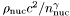], whereand.

Definition at line 376 of file hoteos.h.

 double Lorene::Ideal_gas::m_0 [protected]

Individual particule mass(cf.

Eq. (2)) [unit:].

Definition at line 381 of file hoteos.h.

 string Lorene::Hot_eos::name [protected, inherited]

EOS name.

Definition at line 72 of file hoteos.h.

 Eos* Lorene::Hot_eos::p_cold_eos [mutable, protected, inherited]

Corresponding cold Eos.

Definition at line 108 of file hoteos.h.

 double Lorene::Ideal_gas::unsgam1 [protected]Definition at line 384 of file hoteos.h.

The documentation for this class was generated from the following files:

Generated on 7 Dec 2019 for LORENE by1.6.1